## 5.169. golomb

Origin

Inspired by [Golomb72].

Constraint

$\mathrm{𝚐𝚘𝚕𝚘𝚖𝚋}\left(\mathrm{𝚅𝙰𝚁𝙸𝙰𝙱𝙻𝙴𝚂}\right)$

Argument
 $\mathrm{𝚅𝙰𝚁𝙸𝙰𝙱𝙻𝙴𝚂}$ $\mathrm{𝚌𝚘𝚕𝚕𝚎𝚌𝚝𝚒𝚘𝚗}\left(\mathrm{𝚟𝚊𝚛}-\mathrm{𝚍𝚟𝚊𝚛}\right)$
Restrictions
 $\mathrm{𝚛𝚎𝚚𝚞𝚒𝚛𝚎𝚍}$$\left(\mathrm{𝚅𝙰𝚁𝙸𝙰𝙱𝙻𝙴𝚂},\mathrm{𝚟𝚊𝚛}\right)$ $\mathrm{𝚅𝙰𝚁𝙸𝙰𝙱𝙻𝙴𝚂}.\mathrm{𝚟𝚊𝚛}\ge 0$ $\mathrm{𝚜𝚝𝚛𝚒𝚌𝚝𝚕𝚢}_\mathrm{𝚒𝚗𝚌𝚛𝚎𝚊𝚜𝚒𝚗𝚐}$$\left(\mathrm{𝚅𝙰𝚁𝙸𝙰𝙱𝙻𝙴𝚂}\right)$
Purpose

Given a strictly increasing sequence ${X}_{1},{X}_{2},\cdots ,{X}_{n}$, enforce all differences ${X}_{i}-{X}_{j}$ between two variables ${X}_{i}$ and ${X}_{j}$ $\left(i>j\right)$ to be distinct.

Example
$\left(〈0,1,4,6〉\right)$

Figure 5.169.1 gives a graphical interpretation of the solution given in the example in term of a graph: each vertex corresponds to a value of $〈0,1,4,6〉$, while each arc depicts a difference between two values. The $\mathrm{𝚐𝚘𝚕𝚘𝚖𝚋}$ constraint holds since one can note that these differences 1, 4, 6, 3, 5, 2 are all-distinct.

##### Figure 5.169.1. Graphical representation of the solution $\mathbf{0}$, $\mathbf{1}$, $\mathbf{4}$, $\mathbf{6}$ (differences are displayed in light red and are pairwise distinct).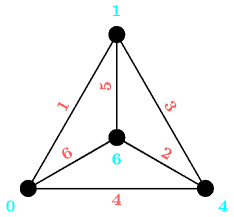Typical
$|\mathrm{𝚅𝙰𝚁𝙸𝙰𝙱𝙻𝙴𝚂}|>2$
Symmetry

One and the same constant can be added to the $\mathrm{𝚟𝚊𝚛}$ attribute of all items of $\mathrm{𝚅𝙰𝚁𝙸𝙰𝙱𝙻𝙴𝚂}$.

Arg. properties

Contractible wrt. $\mathrm{𝚅𝙰𝚁𝙸𝙰𝙱𝙻𝙴𝚂}$.

Usage

This constraint refers to the Golomb ruler problem. We quote the definition from [Shearer96]: “A Golomb ruler is a set of integers (marks) ${a}_{1}<\cdots <{a}_{k}$ such that all the differences ${a}_{i}-{a}_{j}$ $\left(i>j\right)$ are distinct”.

Remark

Different constraints models for the Golomb ruler problem were presented in [SmithStergiouWalsh99].

Algorithm

At a first glance, one could think that, because it looks so similar to the $\mathrm{𝚊𝚕𝚕𝚍𝚒𝚏𝚏𝚎𝚛𝚎𝚗𝚝}$ constraint, we could have a perfect polynomial filtering algorithm. However this is not true since one retrieves the same variable in different vertices of the graph. This leads to the fact that one has incompatible arcs in the bipartite graph (the two classes of vertices correspond to the pair of variables and to the fact that the difference between two pairs of variables takes a specific value). However one can still reuse a similar filtering algorithm as for the $\mathrm{𝚊𝚕𝚕𝚍𝚒𝚏𝚏𝚎𝚛𝚎𝚗𝚝}$ constraint, but this will not lead to perfect pruning.

Counting
 Length ($n$) 2 3 4 5 6 7 8 9 10 11 Solutions 3 2 2 4 8 10 2 2 2 4

Number of solutions for $\mathrm{𝚐𝚘𝚕𝚘𝚖𝚋}$: domains $0..k$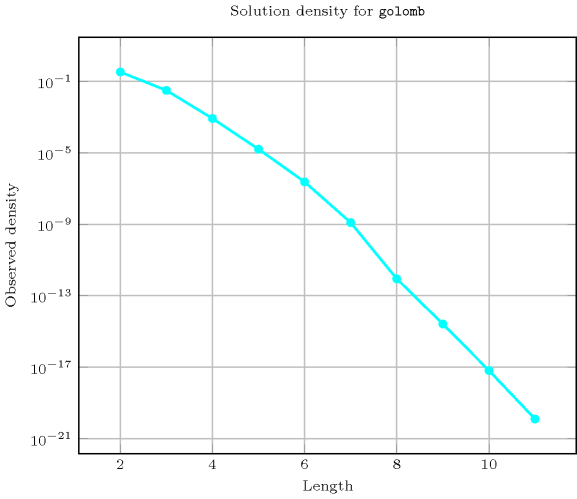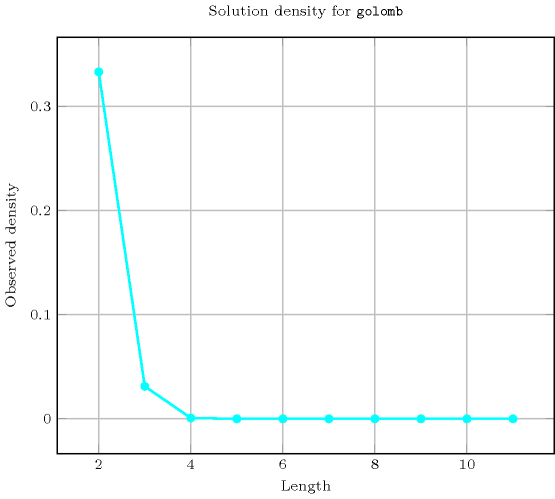Keywords
Cond. implications

$•$ $\mathrm{𝚐𝚘𝚕𝚘𝚖𝚋}\left(\mathrm{𝚅𝙰𝚁𝙸𝙰𝙱𝙻𝙴𝚂}\right)$

implies $\mathrm{𝚒𝚗𝚌𝚛𝚎𝚊𝚜𝚒𝚗𝚐}_\mathrm{𝚗𝚟𝚊𝚕𝚞𝚎}$$\left(\mathrm{𝙽𝚅𝙰𝙻},\mathrm{𝚅𝙰𝚁𝙸𝙰𝙱𝙻𝙴𝚂}\right)$

when  $\mathrm{𝙽𝚅𝙰𝙻}=$$\mathrm{𝚗𝚟𝚊𝚕}$$\left(\mathrm{𝚅𝙰𝚁𝙸𝙰𝙱𝙻𝙴𝚂}.\mathrm{𝚟𝚊𝚛}\right)$.

$•$ $\mathrm{𝚐𝚘𝚕𝚘𝚖𝚋}\left(\mathrm{𝚅𝙰𝚁𝙸𝙰𝙱𝙻𝙴𝚂}\right)$

implies $\mathrm{𝚜𝚘𝚏𝚝}_\mathrm{𝚊𝚕𝚕𝚍𝚒𝚏𝚏𝚎𝚛𝚎𝚗𝚝}_\mathrm{𝚌𝚝𝚛}$$\left(𝙲,\mathrm{𝚅𝙰𝚁𝙸𝙰𝙱𝙻𝙴𝚂}\right)$.

Derived Collection
$\mathrm{𝚌𝚘𝚕}\left(\begin{array}{c}\mathrm{𝙿𝙰𝙸𝚁𝚂}-\mathrm{𝚌𝚘𝚕𝚕𝚎𝚌𝚝𝚒𝚘𝚗}\left(𝚡-\mathrm{𝚍𝚟𝚊𝚛},𝚢-\mathrm{𝚍𝚟𝚊𝚛}\right),\hfill \\ \mathrm{𝚒𝚝𝚎𝚖}\left(𝚡-\mathrm{𝚅𝙰𝚁𝙸𝙰𝙱𝙻𝙴𝚂}.\mathrm{𝚟𝚊𝚛},𝚢-\mathrm{𝚅𝙰𝚁𝙸𝙰𝙱𝙻𝙴𝚂}.\mathrm{𝚟𝚊𝚛}\right)\right]\hfill \end{array}\right)$
Arc input(s)

$\mathrm{𝙿𝙰𝙸𝚁𝚂}$

Arc generator
$\mathrm{𝐶𝐿𝐼𝑄𝑈𝐸}$$↦\mathrm{𝚌𝚘𝚕𝚕𝚎𝚌𝚝𝚒𝚘𝚗}\left(\mathrm{𝚙𝚊𝚒𝚛𝚜}\mathtt{1},\mathrm{𝚙𝚊𝚒𝚛𝚜}\mathtt{2}\right)$

Arc arity
Arc constraint(s)
$\mathrm{𝚙𝚊𝚒𝚛𝚜}\mathtt{1}.𝚢-\mathrm{𝚙𝚊𝚒𝚛𝚜}\mathtt{1}.𝚡=\mathrm{𝚙𝚊𝚒𝚛𝚜}\mathtt{2}.𝚢-\mathrm{𝚙𝚊𝚒𝚛𝚜}\mathtt{2}.𝚡$
Graph property(ies)
$\mathrm{𝐌𝐀𝐗}_\mathrm{𝐍𝐒𝐂𝐂}$$\le 1$

Graph model

When applied on the collection of items $〈\mathrm{𝚅𝙰𝚁}\mathtt{1},\mathrm{𝚅𝙰𝚁}\mathtt{2},\mathrm{𝚅𝙰𝚁}\mathtt{3},\mathrm{𝚅𝙰𝚁}\mathtt{4}〉$, the generator of derived collection generates the following collection of items: $〈\mathrm{𝚅𝙰𝚁}\mathtt{2}\mathrm{𝚅𝙰𝚁}\mathtt{1},\mathrm{𝚅𝙰𝚁}\mathtt{3}\mathrm{𝚅𝙰𝚁}\mathtt{1},\mathrm{𝚅𝙰𝚁}\mathtt{3}\mathrm{𝚅𝙰𝚁}\mathtt{2},\mathrm{𝚅𝙰𝚁}\mathtt{4}\mathrm{𝚅𝙰𝚁}\mathtt{1},\mathrm{𝚅𝙰𝚁}\mathtt{4}\mathrm{𝚅𝙰𝚁}\mathtt{2},\mathrm{𝚅𝙰𝚁}\mathtt{4}\mathrm{𝚅𝙰𝚁}\mathtt{3}〉$. Note that we use a binary arc constraint between two vertices and that this binary constraint involves four variables.

Parts (A) and (B) of Figure 5.169.2 respectively show the initial and final graph associated with the Example slot. Since we use the $\mathrm{𝐌𝐀𝐗}_\mathrm{𝐍𝐒𝐂𝐂}$ graph property we show one of the largest strongly connected components of the final graph. The constraint holds since all the strongly connected components have at most one vertex: the differences 1, 2, 3, 4, 5, 6 that one can construct from the values 0, 1, 4, 6 assigned to the variables of the $\mathrm{𝚅𝙰𝚁𝙸𝙰𝙱𝙻𝙴𝚂}$ collection are all-distinct.

##### Figure 5.169.2. Initial and final graph of the $\mathrm{𝚐𝚘𝚕𝚘𝚖𝚋}$ constraint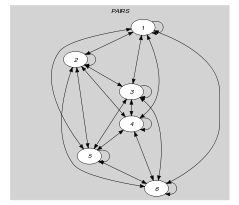(a)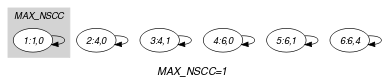(b)